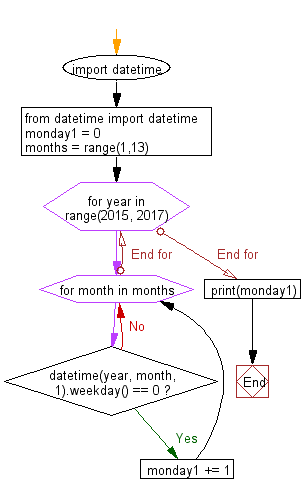﻿ Python: Count the number of Monday of the 1st day of the month between two years - w3resource

# Python: Count the number of Monday of the 1st day of the month between two years

## Python Datetime: Exercise-24 with Solution

Write a Python program to count the number of Mondays on the 1st day of the month from 2015 to 2016.

Sample Solution:

Python Code:

``````import datetime
from datetime import datetime
monday1 = 0
months = range(1,13)
for year in range(2015, 2017):
for month in months:
if datetime(year, month, 1).weekday() == 0:
monday1 += 1
print(monday1)
```
```

Sample Output:

```3
```

Flowchart:Python Code Editor: